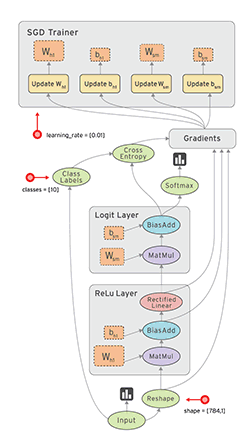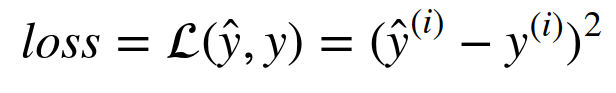{{announcement.body}}
{{announcement.title}}

# TensorFlow in a Nutshell

DZone 's Guide to

# TensorFlow in a Nutshell

### Let's take a look at TensorFlow and explore graphs and the benefits of using them. Also look at what a session is.

· AI Zone ·
Free Resource

Comment (0)

Save
{{ articles.views | formatCount}} Views

TensorFlow is an open-source Machine Learning library for research and production. TensorFlow offers APIs for beginners and experts to develop for desktop, mobile, web, and cloud. See the sections below to get started.

Installing TensorFlow is an easy task unless until you complicated it. The recommended method to install it is using the virtual environment. Python virtual environments are used to isolate package installation from the system.

If you are not worrying about it and using ubuntu just type following command in terminal. It's the easiest way for a lazy person :P

``pip3 install tensorflow``

Before diving into the coding you must know some terms related to Tensorflow.

## What Is a Graph?

TensorFlow uses a data flow graph to represent your computation in terms of the dependencies between individual operations. This leads to a low-level programming model in which you first define the data flow graph, then create a TensorFlow session to run parts of the graph across a set of local and remote devices. This is commonly called a data flow programming model, especially for parallel computing.Nodes in the graph represent mathematical operations, while the graph edges represent the multidimensional data arrays (tensors) communicated between them. The flexible architecture allows you to deploy computation to one or more CPUs or GPUs in a desktop, server, or mobile device with a single API.

You can see the graph as the functions you have used in mathematics. When you plug variables it gives you the output. If the function is called f, this relation is denoted y = f (x), the element x is the argument or input of the function, and y is the value of the function, the output, or the image of x by f. here the function f (x) could be seen as the graph where it 's doing an operation.

## So, What Are the Benefits of Using Graphs?

1. Parallelism: By using explicit edges to represent dependencies between operations, it is easy for the system to identify operations that can execute in parallel.
2. Distributed execution: By using explicit edges to represent the values that flow between operations, it is possible for TensorFlow to partition your program across multiple devices (CPUs, GPUs, and TPUs) attached to different machines. TensorFlow inserts the necessary communication and coordination between devices.
3. Compilation: TensorFlow’s XLA compiler can use the information in your dataflow graph to generate faster code, for example, by fusing together adjacent operations.
4. Portability: The dataflow graph is a language-independent representation of the code in your model. You can build a dataflow graph in Python, store it in a SavedModel, and restore it in a C++ program for low-latency inference.

## What Is a Session?

I’ve seen a lot of confusion over the rules of tf.Graph and tf.Session in TensorFlow.It’s simple:

A graph defines the computation. It doesn’t compute anything, It doesn’t hold any values, it just defines the operations that you specified in your code.
A session allows executing graphs or part of graphs. It allocates resources (on one or more machines) for that and holds the actual values of intermediate results and variables.

A Session object encapsulates the environment in which Operation objects are executed, and Tensor objects are evaluated. Which means that none the operators and variables defined in the graph-definition part are being executed. until the session is executed.

### Writing and Running Programs in TensorFlow Has the Following Steps:

1. Create Tensors (variables) that are not yet executed/evaluated.
2. Write operations between those Tensors.
4. Create a Session.
5. Run the Session.

In order to make you guess for the rest of the article, I am giving a TensorFlow program which will calculate the error in a linear graph.

Here, I define the function:``````y_hat = tf.constant(36, name='y_hat')            # Define y_hat constant. Set to 36.
y = tf.constant(39, name='y')                    # Define y. Set to 39

loss = tf.Variable((y - y_hat)**2, name='loss')  # Create a variable for the loss

init = tf.global_variables_initializer()         # When init is run later (session.run(init)),
# the loss variable will be initialized and ready to be computed
with tf.Session() as session:                    # Create a session and print the output
session.run(init)                            # Initializes the variables
print(session.run(loss))                     # Prints the loss``````

You will end up getting 9 in the console. So, the first two lines describe that we are defining two constants. Then, using the sensor flow variable, we define the loss function. When we created a variable for the loss, we simply defined the loss as a function of other quantities but did not evaluate its value. To evaluate it, we had to run `init=tf.global_variables_initializer()` . That initialized the loss variable, and in the last line, we were finally able to evaluate the value of loss and print its value. We then get the output as we created the session and executed it.

## Constants vs. Variables

In TensorFlow, the differences between constants and variables are that when you declare some constant, its value can't be changed in the future (also the initialization should be with a value, not with the operation).

Nevertheless, when you declare a Variable, you can change its value in the future with tf.assign() method (and the initialization can be achieved with a value or operation).

The function tf.global_variables_initializer() initializes all variables in your code with the value passed as the parameter, but it works in async mode, so doesn't work properly when dependencies exist between variables.

Now let us look at an easy example. Run the cell below:

``````a = tf.constant(2)
b = tf.constant(10)
c = tf.multiply(a,b)
print(c)

As expected, you will not see 20! You got a tensor saying that the result is a tensor ( `Tensor("Mul:0", shape=(), dtype=int32)` ) that does not have the shape attribute, and is of type "int32". All you did was put in the "computation graph," but you have not run this computation yet. In order to actually multiply the two numbers, you will have to create a session and run it.

``````sess = tf.Session()
print(sess.run(c))

Great! To summarize, remember to initialize your variables, create a session, and run the operations inside the session.

## What Is a Placeholder?

A placeholder is an object whose value you can specify only later. To specify values for a placeholder, you can pass in values by using a "feed dictionary" (`feed_dict` variable). Below, we created a placeholder for x. This allows us to pass in a number later when we run the session.

``````x = tf.placeholder(tf.int64, name = 'x')
print(sess.run(2 * x, feed_dict = {x: 3}))
sess.close()

When you first defined `x` you did not have to specify a value for it. A placeholder is simply a variable that you will assign data to only later when running the session. We say that you feed data to these placeholders when running the session.

## Here's What's Happening

When you specify the operations needed for a computation, you are telling TensorFlow how to construct a computation graph. The computation graph can have some placeholders whose values you will specify only later. Finally, when you run the session, you are telling TensorFlow to execute the computation graph.

There are two typical ways to create and use sessions in TensorFlow:

Method 1:

``````sess = tf.Session()
# Run the variables initialization (if needed), run the operations
result = sess.run(..., feed_dict = {...})
sess.close() # Close the session``````

Method 2:

``````with tf.Session() as sess:
# run the variables initialization (if needed), run the operations
result = sess.run(..., feed_dict = {...})
# This takes care of closing the session for you :)``````

If you run the `with tf.Session() as sess:` then you don't need to close the session. This takes care of closing the session for you :)

let's look defining a sigmoid function. Tensorflow offers a variety of commonly used neural network functions like tf.sigmoid and tf.softmax. For an exercise, let's code the sigmoid function.

``````# FUNCTION: sigmoid

def sigmoid(z):
"""
Computes the sigmoid of z

Arguments:
z -- input value, scalar or vector

Returns:
results -- the sigmoid of z
"""

### START CODE HERE ### ( approx. 4 lines of code)
# Create a placeholder for x. Name it 'x'.
x = tf.placeholder(tf.float32, name="x")

# compute sigmoid(x)
sigmoid = tf.sigmoid(x)

# Create a session, and run it. Please use the method 2 explained above.
# You should use a feed_dict to pass z's value to x.
with tf.Session() as sess:
# Run session and call the output "result"
result = result = sess.run(sigmoid, feed_dict = {x: z})

### END CODE HERE ###

return result

print ("sigmoid(0) = " + str(sigmoid(0)))
print ("sigmoid(12) = " + str(sigmoid(12)))

# sigmoid(0) = 0.5
# sigmoid(12) = 0.999994``````

• Tensor flow and how it works
• Graphs and sessions
• Variables and Constants
• Create placeholders
• Specify the computation graph corresponding to operations you want to compute
• Create the session
• Run the session, using a feed dictionary if necessary to specify placeholder variables' values.

In the next article, I will meet you with, "How to Create a Neural Network Using TensorFlow." Thanks for reading!

Topics:
ai ,ai graphs ,deeplearning ,ml library ,python ,tensorflow

Comment (0)

Save
{{ articles.views | formatCount}} Views

Opinions expressed by DZone contributors are their own.